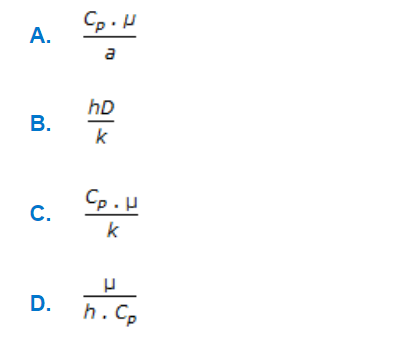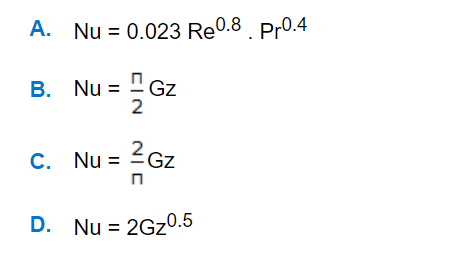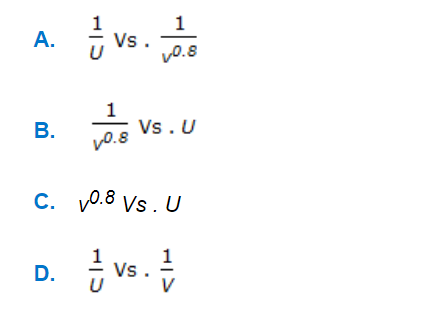# E-PolyLearning

 21. Prandtl number is given bya. A b. B c. C d. D

 22. For a liquid in laminar flow through a very long tube, when the exit fluid temperature approaches the wall temperature, the equation to be used isa. A b. B c. C d. D
 23. A graph between __________ is called Wilson plot.a. A b. B c. C d. D
 24. What is the geometric mean of two heat transfer areas A1 and A2 ?a. A b. B c. C d. D